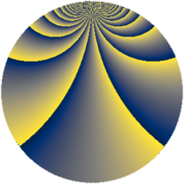# Properties

 Label 900.3.tLevel $900$ Weight $3$ Character orbit 900.t Rep. character $\chi_{900}(151,\cdot)$ Character field $\Q(\zeta_{6})$ Dimension $444$ Sturm bound $540$

# Related objects

## Defining parameters

 Level: $$N$$ $$=$$ $$900 = 2^{2} \cdot 3^{2} \cdot 5^{2}$$ Weight: $$k$$ $$=$$ $$3$$ Character orbit: $$[\chi]$$ $$=$$ 900.t (of order $$6$$ and degree $$2$$) Character conductor: $$\operatorname{cond}(\chi)$$ $$=$$ $$36$$ Character field: $$\Q(\zeta_{6})$$ Sturm bound: $$540$$

## Dimensions

The following table gives the dimensions of various subspaces of $$M_{3}(900, [\chi])$$.

Total New Old
Modular forms 744 468 276
Cusp forms 696 444 252
Eisenstein series 48 24 24

## Trace form

 $$444q + q^{2} + q^{4} - 5q^{6} - 14q^{8} + 8q^{9} + O(q^{10})$$ $$444q + q^{2} + q^{4} - 5q^{6} - 14q^{8} + 8q^{9} - 14q^{12} + 2q^{13} - 38q^{14} - 3q^{16} - 16q^{17} - 20q^{18} + 14q^{21} + 9q^{22} + 83q^{24} + 40q^{26} + 36q^{28} + 26q^{29} + 61q^{32} + 2q^{33} - 5q^{34} - 5q^{36} + 8q^{37} + 93q^{38} + 54q^{41} + 12q^{42} + 258q^{44} + 80q^{46} - 183q^{48} + 1304q^{49} - 40q^{52} - 136q^{53} - 49q^{54} + 86q^{56} + 108q^{57} + 38q^{58} - 6q^{61} + 408q^{62} - 254q^{64} - 468q^{66} - 103q^{68} + 46q^{69} + 21q^{72} + 32q^{73} + 304q^{74} - 33q^{76} + 150q^{77} - 176q^{78} - 32q^{81} + 194q^{82} - 80q^{84} - 25q^{86} - 75q^{88} + 104q^{89} + 378q^{92} + 230q^{93} + 154q^{94} - 416q^{96} - 58q^{97} - 158q^{98} + O(q^{100})$$

## Decomposition of $$S_{3}^{\mathrm{new}}(900, [\chi])$$ into newform subspaces

The newforms in this space have not yet been added to the LMFDB.

## Decomposition of $$S_{3}^{\mathrm{old}}(900, [\chi])$$ into lower level spaces

$$S_{3}^{\mathrm{old}}(900, [\chi]) \cong$$ $$S_{3}^{\mathrm{new}}(36, [\chi])$$$$^{\oplus 3}$$$$\oplus$$$$S_{3}^{\mathrm{new}}(180, [\chi])$$$$^{\oplus 2}$$